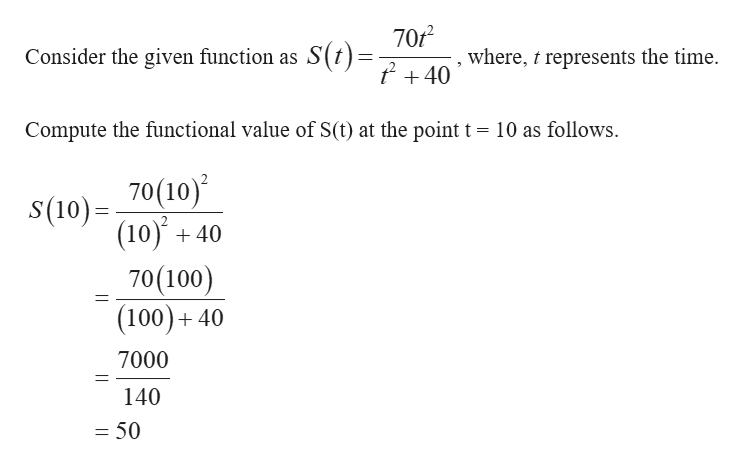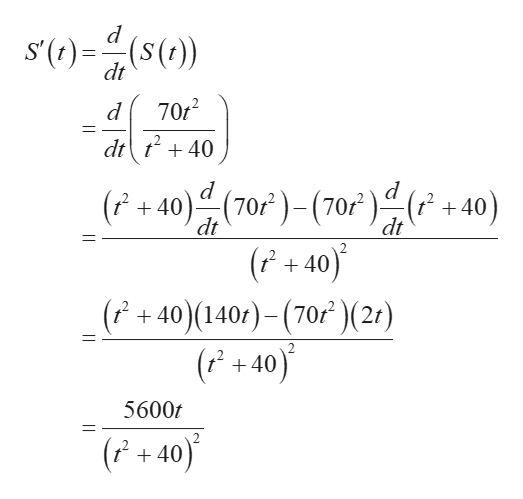# The total sales S(t) (in thousands of CDs) for a compact disc is given by the functionS(t) = (70t2) / (t2+40)Where t is the number of months since the release of the CD. (Note: This number does not include copies distributed by Napster.) Find S(10) and S'(10).S(10) = ?S'(10) = ?

Question
81 views

The total sales S(t) (in thousands of CDs) for a compact disc is given by the function

S(t) = (70t2) / (t2+40)

Where t is the number of months since the release of the CD. (Note: This number does not include copies distributed by Napster.) Find S(10) and S'(10).

S(10) = ?

S'(10) = ?

check_circle

Step 1help_outlineImage Transcriptionclose70f2 Consider the given function as S(t)= +40 where, t represents the time. Compute the functional value of S(t) at the point t = 10 as follows \$(10)70(10) (10) +40 70(100) (100)+ 40 11 7000 140 = 50 fullscreen
Step 2

Calculate the derivative of the function S(t) with r...help_outlineImage Transcriptionclosed s'(f)(()) dt 70r2 d 1 dtt40 d (F+40)(70)(70)(f+40) (P+40) (F +40) (140r)-(70)(2?) (P+40) dt dt 7012 5600t (P+ 40) fullscreen

### Want to see the full answer?

See Solution

#### Want to see this answer and more?

Solutions are written by subject experts who are available 24/7. Questions are typically answered within 1 hour.*

See Solution
*Response times may vary by subject and question.
Tagged in

### Other